#Quality Digest

Featured Product
This Week in Quality Digest Live
Six Sigma Features
Donald J. Wheeler
Using process behavior charts in a clinical setting
Alan Metzel
Introducing the Enhanced Perkin Tracker
Donald J. Wheeler
What you think you know may not be so
William A. Levinson
The AIAG offers a clearly defined and powerful synergy between the three
Donald J. Wheeler
How does it compare with a process behavior chart?
Six Sigma News
Sept. 28–29, 2022, at the MassMutual Center in Springfield, MA
Elsmar Cove is a leading forum for quality and standards compliance
Is the future of quality management actually business management?
Too often process enhancements occur in silos where there is little positive impact on the big picture
Collect measurements, visual defect information, simple Go/No-Go situations from any online device
Good quality is adding an average of 11 percent to organizations’ revenue growth
Floor symbols and decals create a SMART floor environment, adding visual organization to any environment
A guide for practitioners and managers
Making lean Six Sigma easier and adaptable to current workplacesBio

Six Sigma

## Process Behavior Charts for Non-Normal Data, Part 2

### What happens to the range chart?

Published: Tuesday, February 3, 2015 - 16:41

Whenever the original data pile up against a barrier or a boundary value, the histogram tends to be skewed and non-normal in shape. Last month in part one we found that this doesn’t appreciably affect the performance of process behavior charts for location. This month we look at how skewed data affect the charts for dispersion.

In practice, we get appreciable skewness only when the distance between the average and the boundary condition is less than two standard deviations. A careful inspection of the six distributions shown in figure 1 will show that this corresponds to those situations where the skewness is in the neighborhood of 0.90 or larger. When the skewness is smaller than this, the departure for normality is minimal, as may be seen with distribution number 15 in figure 1.

The usual formulas for finding limits for a range chart involve the scaling factors known as D3 and D4.

Lower Range Limit = D3 × Average Range
Upper Range Limit = D4 × Average Range

These scaling factors depend on the bias correction factors, d2 and d3.As outlined in part one, the traditional values for these bias correction factors were computed using a normal distribution for the original data. About 48 years ago Irving Burr finally found the values for the bias correction factors, using 27 different non-normal distributions. Six of these distributions are shown in figure 1.

As the distributions become more skewed, the central portions of each non-normal distribution will become more concentrated. This concentration will result in a slight reduction in the average value for the distribution of the subgroup ranges. Since d2 characterizes the mean of the distribution of subgroup ranges, we should expect the non-normal d2 values to be slightly smaller than the normal theory values, and this is exactly what we see in figure 2.

On the other hand, the elongated tails of the non-normal distributions should create a few more extreme values for the subgroup ranges. These extreme ranges should slightly increase the variation in the distributions of the subgroup ranges. Since d3 characterizes the dispersion of the distribution of subgroup ranges, we should expect the non-normal d3 values to be slightly larger than the normal theory values, and this is exactly what we see in figure 2. Thus, the departures seen in figure 2 are exactly what we should have expected. The question is how much do these departures affect the computation of the limits for the range chart?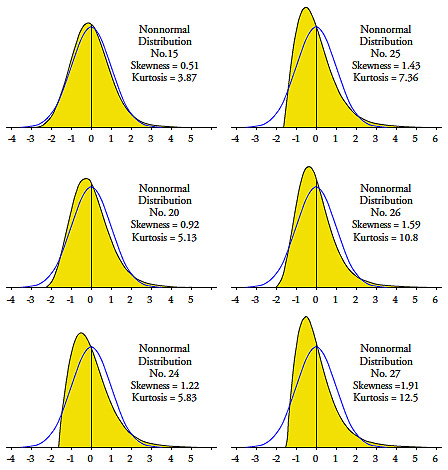Figure 1: Six of the 27 non-normal distributions Burr used

### Burr’s approach

Irving Burr’s original idea was that we could use the values in figure 2 to sharpen up the limits by first computing the skewness and kurtosis statistics for our data and then choosing appropriate bias correction factors from his table. (This is equivalent to today’s practice of letting your software fit a probability model to your data.)

Unfortunately, in practice, the uncertainty in both the skewness and kurtosis statistics is so great that we can never be sure that our estimates for these shape parameters are even remotely correct. Regardless of how many data you have, you will always know more about location and dispersion than you will ever know about skewness and kurtosis. Until you have thousands of data collected from a predictable process, any use of the skewness and kurtosis statistics is an exercise in fitting noise. This inherent and unavoidable problem undermines Burr’s approach.Figure 2: Burr’s values of d2 and d3 for 27 non-normal distributions

So, even though Burr’s idea does not quite work out as intended in practice, we can use the values in figure 2 to assess the effects of non-normality on the computation of the range chart limits. As we did last month, we look at the bias correction factors as unknown variables that must be approximated. With this change in perspective, the question becomes one of assessing the impact of not knowing the exact value for these fundamental constants.

We begin by observing that the scaling factors D3 and D4 involve d3 divided by d2. Since this ratio accentuates the slight changes in location and dispersion seen in figure 2, we compute these ratios in figure 3. Then, for each column in figure 3 we compute the coefficient of variation. These coefficients of variation will summarize how our not knowing the exact values for d2 and d3 will affect the computation of the range chart limits.

Last month we looked at how uncertainty in the bias correction factors affected the limits for charts for location. There we found coefficients of variation in the neighborhood of 2 percent. In figure 3 we find coefficients of variation ranging from 5 percent to 13 percent. So clearly the limits for the range chart are not as robust as the limits for charts for location.

As we saw last month, the uncertainty shown in figure 3 is not the only source of uncertainty in the limits. We need to also consider the uncertainty due to the use of the average range in the computation. Recalling that the coefficient of variation for the average range is the inverse of the square root of twice the number of degrees of freedom, we can do some computations.Figure 3: Uncertainties in scaling factors for range chart limits

Consider an XmR chart based on k = 50 data. The average moving range will have approximately 0.62 (k–1) = 30 degrees of freedom, which results in a coefficient of variation of 12.9 percent. Thus, when we combine the CV values for our two sources of uncertainty, we find that the uncertainty in the limits for the range chart will be, at most: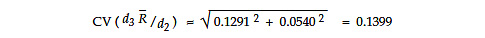While the impact of the uncertainty in the bias correction factors is larger here than it is for the X chart, the dominant source of uncertainty is still the uncertainty in the average range statistic, rather than the uncertainty due to not having exact values for the computation. Whether the uncertainty in the upper range limit is 13 percent or 14 percent will not greatly affect the interpretation of your XmR chart.

For an average and range chart based on k = 25 subgroups of size n = 2, the limits will have about 0.9 k (n–1) = 22 degrees of freedom, so the CV for the average range will be about 15.1 percent and the uncertainty in the upper limit for the range chart will be, at most: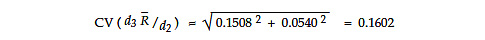Once again, while the impact of the uncertainty in the bias correction factors is larger here than it was with the average chart, it is still not appreciable. Whether the uncertainty in the upper range limit is 15 percent or 16 percent will not greatly affect the interpretation of your average and range chart.

When we combine the uncertainty in the average range with the uncertainty introduced by not knowing the exact values for the bias correction factors, we get the curves shown in figure 4.Figure 4: How variation in both bias correction factors affects limits for ranges

So, how many degrees of freedom will we need in our baseline before the two sources of uncertainty in the range chart limits reach parity? We can use the last row of figure 3 and the formula relating degrees of freedom to the coefficient of variation to obtain the values in figure 5.

 Limits for Ranges Subgroup size 2 3 4 5 8 10 Degrees of freedom 171 104 74 55 36 29

Figure 5: Baseline degrees of freedom needed for parity between uncertainties in range limits

So what can we say based on figures 4 and 5? Initially, when the degrees of freedom are small, there is little need to be concerned about your range chart limits. (Here I would define “small” as less than half the values shown in figure 5.) The dominant source of variation in the limits will be the uncertainty in the average range statistic, and fine-tuning the computation of the upper range limit will add virtually nothing to the interpretation and use of the charts.

Also, as long as there are signals that your process is being operated unpredictably, any questions about the limits will be moot. With an unpredictable process the emphasis needs to be on finding and removing the effects of the assignable causes of unpredictable operation. Since nine processes out of 10 are operated unpredictably, this will remove the sting of having some lack of robustness for the upper range chart limit. (When you have an abundance of real signals, a few false alarms on the range chart will not matter.) Moreover, since 90 percent of your signals of unpredictable behavior will occur on the chart for location, and since signals on the range chart are commonly accompanied by signals on the chart for location, we are not likely to be misled by some lack of robustness for the upper range chart limit. Most of the potential signals you will find with your process behavior chart will be real.

So when do you need to be concerned about the upper range limit? If you have a process where the original data pile up against a boundary or barrier condition, and if that process appears to be operating in a reasonably predictable manner, then you might want to fine-tune the upper range chart limit. But how might we go about doing this when we can’t, in practice, reliably identify a particular probability model to use? A clue on how to proceed is found in figure 6, where the values in figure 3 are plotted vs. the skewness parameters for the different distributions.

In figure 6 we see how the skewness of the distribution affects the computation of the upper range chart limit. The initial point for each curve shows the normal theory value for the ratio of d3 to d2. The horizontal lines attached to these initial values show the value of the traditional calculation for the three-sigma upper range limit. The vertical distances between the plotted points and the horizontal lines will reveal the extent to which the traditional three-sigma upper range limit will be too small for each of the 27 non-normal distributions.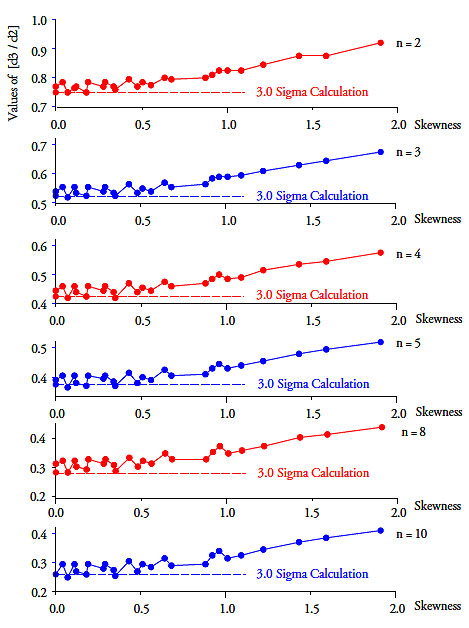Figure 6: The ratios of figure 3

Until the skewness exceeds 0.90, the points of figure 4 tend to cluster in a horizontal band only slightly above the traditional normal theory value. But when the skewness exceeds 0.90 the curves tend to slope upward. This suggests that it is only when we have pronounced skewness that any adjustment is actually needed in the computations of the upper range chart limit. From figure 2 we see that we will have pronounced skewness only when the average falls within two standard deviations of the barrier or boundary condition.

So, if you have a reasonably predictable process where the distance from a barrier or boundary condition to the process average is less than twice the within-subgroup estimate of the standard deviation parameter, then you may wish to inflate the upper range limit to avoid a slightly increased false alarm rate.

But how much do we inflate the upper range limit? To identify an exact value for the ratio of d3 to d2, we would need to pick out a specific distribution. Since we will never have sufficient data to do this in practice, and since an approximate solution is all that we need, we choose to inflate the upper limit by a fixed amount based on the subgroup size. As we can see in figure 7, for n = 2, a computed upper 3.7 sigma limit on the range chart will be sufficiently conservative to handle even the most skewed of Burr’s distributions. For n = 3, a computed 3.8 sigma upper range limit will be sufficiently conservative to work for most of Burr’s distributions. For n = 4 compute an upper 3.9 sigma limit on the range chart. For n = 5 compute an upper 4.0 sigma limit on the range chart. For n = 10 compute an upper 4.5 sigma limit on the range chart. By increasing the computed upper range limit by 0.1 sigma for each unit increase in the subgroup size, we obtain reasonably conservative approximations for the actual three-sigma upper range limits that will work with heavily skewed data.

We should note that the adjustments given here are merely adjustments to the computations to allow for the fact that when the original data are excessively skewed, the distributions for the subgroup ranges will also become more skewed. The adjusted upper range limits are still approximate three-sigma limits even though they are computed like they are 3.7 to 4.5 sigma limits.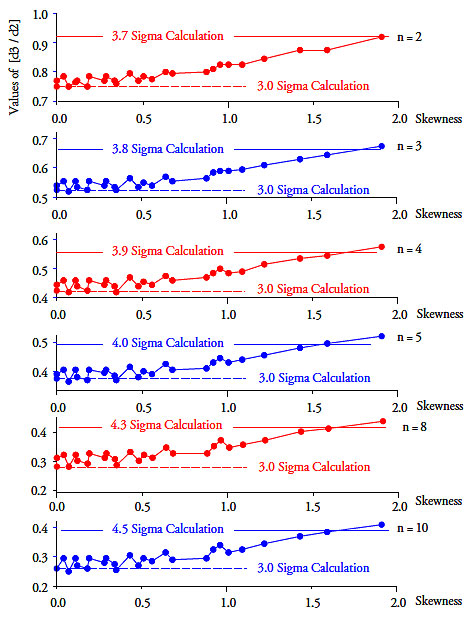Figure 7: Adjusting the upper range limit for a predictable but skewed process

By computing upper range limits in keeping with the guideline shown in figure 7, you can minimize the occurrence of false alarms on the range chart even when the original data are severely skewed. Since the bulk of true signals will occur on the charts for location, either with or without accompanying signals on the range chart, this adjustment to the computations is not needed until after the process has been operated in a reasonably predictable manner.

### So what do we do in practice?

Remember, the objective is to take the right action. The computations are merely a means to help characterize the process behavior. The objective is not to compute the right number, nor to find the best estimate of the right value, nor to find limits that correspond to a specific alpha-level. You only need limits that are good enough to allow you to separate the potential signals from the probable noise so you can take the right action. The limits on a process behavior chart are a statistical axe: They work by brute force. Just as there is no point in putting too fine of an edge on an axe, we also do not need high precision when we calculate our limits. The generic three-sigma limits of a process behavior chart are sufficient to separate dominant cause-and-effect relationships from the run-of-the-mill routine variation. This is why you can take the right action without having to specify a reference distribution, or waiting until you have some magic number of degrees of freedom.

In practice, nine times out of 10, your signals will be found on the chart for location. It is rare indeed to find signals on a range chart without accompanying signals on the chart for location. Thus, in practice we generally give more emphasis to the charts for location. This is appropriate. And as we found in part one, we don’t need to know the exact value for d2 in order for our charts for location to work.

So while Irving Burr built a more complex mousetrap, the difficulties of using his approach in practice make it less useful than the traditional approach. Instead of fine-tuning the bias correction factors to make small adjustments to the limits on a process behavior chart, it is simpler, easier, and better to use the traditional scaling factors to compute the limits. This will not only save you from becoming lost in the details of the computations, but also allow you to get on with the job of discovering the assignable causes that are preventing your process from operating up to its full potential.

If your process shows signals of exceptional variation on the chart for location, then do not attempt to assess the skewness of the histogram. When your process is going on walkabout, the histogram doesn’t represent a single process but many different processes piled up together. In this case any skewness of the histogram doesn’t represent any inherent property of the process, but rather characterizes the mixed-up nature of the process outcomes. By far, the most common cause of a skewed histogram is a process going on walkabout.

If your process appears to be operating predictably based on the chart for location, and if the original data pile up near a boundary condition or barrier in such a way that the average is within two standard deviations of the boundary value (based on a within-subgroup measure of dispersion), then you might want to adjust the upper range chart limit upward according to the guideline shown in figure 7 in order to avoid false alarms on the range chart due to the effects of skewness.

If the original data don’t have the required amount of skewness, no adjustment is needed. (This corresponds to a histogram from a predictable process having an average that is more than two sigma units away from a barrier or boundary condition.)

The best analysis is the simplest analysis that allows you to discover what you need to know. And in this regard, the simple process behavior chart with its three-sigma limits computed using the traditional scaling factors is the undisputed champion. For those situations where the process appears to be operated predictably and yet the data are seriously skewed, a simple adjustment in how we compute the upper range limit can minimize false alarms without unnecessary complexity.

### Discuss### Donald J. Wheeler

Find out about Dr. Wheeler’s virtual seminars for 2023 at www.spcpress.com. Dr. Wheeler is a fellow of both the American Statistical Association and the American Society for Quality who has taught more than 1,000 seminars in 17 countries on six continents. He welcomes your questions; you can contact him at djwheeler@spcpress.com.

### WE rules on XmR charts of non-normal individual values

William!

If the WE rules are based on the normal distribution, does this imply that the statement "Normality is not a pre-requisite for a process behavior chart" is valid for only WE Rule 1 (a data point outside the 3 sigma limits)? How would you then treat the other WE rules in control charts of individual values (XmR)?

### X chart as worst case

Since the central limit theorem doesn't help for charts for individuals, the WE rules will work very poorly for non-normal systems. The ideal would be to set the zone limits at the quantiles of the actual distribution that correspond to the 1 and 2 sigma limits for a normal distribution.

If we had some actual data, I could show how this works.

### Non-normality of data and all WE Rules

Both Parts 1 and 2 of Dr. Wheeler  explain that non-normality of data  is not critical for the primary purpose of control charting: to decide when a process is out of control and take action about the behavior of the process

Normal
0

false
false
false

EN-US
X-NONE
HE

However, "Action" is also taken not only when  a data point exceeds the 3 sigma limits (Detection Rule 1) but also when one of a Run Tests (Western Electric Rules) fails.  To complete the picture, I would like Dr. Wheeler to also address how departure from normality affects the validity of the other Detection Rules 2, 3 and 4, which are also used to evaluate the begavior of a process.

### Effect on Western Electric Rules

The Zone C test (8 consecutive points above or below the center line) relies on the assumption that the center line is the median (50:50 chance if the process is in control). This is true when the distribution is normal. It is emphatically not true when the distribution is non-normal.

The risks for Zones A and B also are calculated under the assumption of normality. I would expect the false alarm risks to be significantly higher when the distribution is skewed, although of course the problem won't be as bad for sample averages due to the central limit theorem.

My book on SPC for Real-World Applications uses as an example a gamma distribution for which the chance of exceeding the 3-sigma UCL is 0.01034, or more than seven times as great as what we expect (0.00135). This is not a show-stopper if you are not worried about false alarms, but it is definitely an issue when you calculate Ppk. This can be off by orders of magnitude in terms of the nonconforming fraction. You can, in fact, have a centered "Six Sigma" process that gives you 93 DPMO, or 93,000 times the expected one per billion.

### Another myth: the "actual" distribution

ADB's recommendation is a great one; that's a book that--frankly--if you haven't read it, you probably shouldn't enter into discussions on normality and the process behavior chart.

A more fundamental point, though, is the problem of trying to assume that there is an "actual distribution" and that you can use it to estimate with any precision the fraction non-conforming. While it is sometimes a useful exercise to estimate that fraction, it should always be presented with a lot of caveats; additionally, with every decimal point you get further from sound and reasonable conjecture in your prediction and closer to that cliff over which you fall into the land of pure and unadulterated fantasy.

To Tony's point, I have a package for Modeling and Simulation with very powerful curve-fitting engine. I can assess the goodness of fit of hundreds of distributions, comparing four different fit tests, resulting in parameter estimates out to 8 decimal points. While this is impressive (at least to me) and often useful in simulations, I wouldn't consider using it for SPC. I do, however, always try to use data that exhibit some reasonable evidence of a state of statistical control before I try to fit a distribution for further use as a modeling and simulation assumption. There can be no assumption of any distribution without a state of statistical control.

What Don has done with these articles is to provide us with a very reasonable and practical approach to adjusting the action limits when we know we have data that naturally appear skewed.

### Normality and the Process Behaviour Chart

I would suggest that Dr Wheeler's little book "Normality and the Process Behaviour Chart" is far better value than wasting money on products like Minitab in a vain attempt to plot probability distributions and to attempt to normalize data.

### It is mandatory to use the actual distribution for Ppk

http://www.qualitydigest.com/inside/six-sigma-column/making-decisions-no... discusses the central limit theorem. The truth is that, if you have a big enough sample, your sample averages will behave as if they come from a normal distribution regardless of the underlying distribution--even one as egregiously non-normal as the exponential distribution. Ranges might be another matter, but the distribution of ranges also becomes more normal as the sample size increases.

You must, however, use the actual underlying distribution to calculate the nonconforming fraction, and therefore the Ppk you quote to your customers. The Automotive Industry Action Group's SPC manual provides two ways to do this, but both require calculation of the quantiles from the underlying distribution. My preference is simply to calculate the fraction outside the specificatiohn limit, e.g. 1-F(UCL) where F is the cumulative distribution.

The other method cited by the AIAG is PP = (USL-LSL)/(Q(0.99865)-Q(0.00135)) where Q is the quantile of the underying distribution. If this distribution is normal, of course, the formula becomes the familiar (USL-LSL)/(6 sigma). (The problem is that this approach doesn't work for PPU and PPL, and therefore not for PPk.) If it is not normal, you must fit the underlying distribution to get Q(0.99865) and Q(0.00135).

Since you MUST fit your data to the underlying distribution to determine Ppk, you have already done the work necessary to set control limits for the actual distribution, which means there is no practical reason to not do it that way.

Thanks for the recommendation.

### R charts with exact control limits

If the underlying distribution is known (e.g. as tested with goodness of fit tests), it is possible to set control limits for the R chart with known false alarm risks. Skewness and kurtosis are essentially useless for this, and Dr. Wheeler's article reinforces this perception. (I have personally not used skewness or kurtosis for anything since learning about them in night school.) The correct approach is to fit the distribution parameters via a maximum likelihood method. Minitab and StatGraphics will do this for a wide variety of distributions such as the Weibull and gamma distributions.

Wilks, S.S. 1948. "Order Statistics." Bulletin of the American Mathematical Society 54 (1948), Part 1:6-50 then provides an equation for the distribution of the range of the distribution in question. You can then calculate, for example, the upper 0.99865 quantile of the range, which gives the same false alarm risk as a 3-sigma Shewhart chart. This IS computationally challenging for anything but an exponential distribution, though, because StatGraphics and Minitab don't do it. My book on SPC for non-normal distribution shows how to do the job with numerical integration in Visual Basic for Applications, and I was able to reproduce tabulated quantiles of ranges from a normal distribution.

These ranges are NOT normally distributed. For a sample of 4, the upper 0.99865 quantile of the range from a normal distribution is 5.20 and not 4.698 (the tabulated D2 factor) times the standard deviation. As the sample size increases, though, the range becomes more and more normally distributed.

As stated in the article, "The objective is not to compute the right number, nor to find the best estimate of the right value, nor to find limits that correspond to a specific alpha-level." The normal approximation is good enough in many situations, e.g. if the false alarm risk is really 0.00150 rather than 0.00135, this is not going to make a real difference on the shop floor. If we use D2 = 4.698 rather than 5.20 for a sample of 4, the higher false alarm risk is not likely to be a real (practical) problem.

If, on the other hand, the false alarm risk is 0.027 rather than 0.00135 (20 times the expected risk), and I can provide an example using the range of a sample of 4 from an exponential distribution, the production workers are going to wonder why they are chasing so many false alarms. This, at best, wastes their time (muda). Matters become worse if the false alarms result in overadjustment.

Again, my results depend on fitting the actual distribution, which is likely to be known based on the nature of the process, as opposed to artificial models that rely on skewness and kurtosis, or other approaches such as the Johnson distributions that, while they might provide a good fit for the data, don't have a real relationship to the underlying process. As an example, impurities (undesirable random arrivals) are likely to follow a gamma distribution. This can be confirmed with goodness of fit tests after one fits the distribution to the data.

All bets are, of course, off if the process is not predictable (under control) because, regardless of what distribution is correct for the data, the parameters are going to be based on bimodal (or even worse) data.